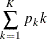Table Output

For each design, the SEQDESIGN procedure displays the Design Information, Method Information, and Boundary Information tables by default.

Boundary Information

The Boundary Information table displays the following information at each stage:

• proportion of information

• actual information level, if the maximum information is either specified or derived

• alternative references with the specified statistic scale. If a p-value scale is specified, the standardized Z scale is used.

• boundary values with the specified statistic scale to reject or accept the null hypothesis

Note that implicitly, the boundary information table also contains variables for the boundary scale, stopping criterion, and type of alternative hypothesis. That is, if an ODS statement is used to save the table, the data set also contains the variables _Scale_ for the boundary scale, _Stop_ for the stopping criterion, and _ALT_ for the type of alternative hypothesis.

The Design Information table displays the design specifications and derived statistics. The derived Max Information (Percent Fixed-Sample) is the maximum information for the sequential design in percentage of the corresponding fixed-sample information.

The Null Ref ASN (Percent Fixed-Sample) is the average sample number (expected sample size for nonsurvival data or expected number of events for survival data) required under the null hypothesis for the group sequential design in percentage of the corresponding fixed-sample design. Similarly, the Alt Ref ASN (Percent Fixed-Sample) is the average sample number required under the alternative reference for the group sequential design in percentage of the corresponding fixed-sample design.

If both the maximum information (MAXINFO= option) and the alternative reference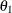(ALTREF= option) are specified, then either the ALPHA= option is used to derive the Type II error probability(BOUNDARYKEY=ALPHA) or the BETA= option is used to derive the Type I error probability(BOUNDARYKEY=BETA).

Error Spending Information

The Error Spending Information table displays the following information at each stage:

• proportion of information

• actual information level, if the maximum information is either specified or derived

• cumulative error spending for each boundary

Method Information

The Method Information table displays detailed method information for the design. For each boundary, it displays the following:

• the group sequential method used

• theorerrors

• the specified parameter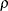, if an error spending function is used

• the specified parametersandwith the derived critical value C, if a unified family method is used

• the alternative reference, if either the ALTREF= or the MAXINFO= option is specified

• the derived drift parameter,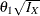, where
is the maximum information andis the alternative reference

Note that the alternative references are displayed with the MLE scale in the Method Information table. In contrast, the alternative references in the Boundary Information table are displayed with the specified statistic scale (if the p-value scale is not specified) or the standardized Z scale (if the p-value scale is specified).

Powers and Expected Sample Sizes

The Powers and Expected Sample Sizes table displays the following information under each of the specified hypothetical references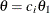, whereis the alternative reference and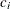are values specified in the CREF= option.

• coefficientfor the hypothetical references. The value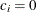corresponds to the null hypothesis and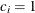corresponds to the alternative hypothesis

• power

• expected sample size, as percentage of fixed-sample size

For a one-sided design, the power and expected sample sizes under the hypothetical referencesare displayed.

For a two-sided symmetric design, the power and expected sample sizes under each of the hypothetical references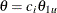are displayed, where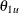is the upper alternative reference.

For a two-sided asymmetric design, the power and expected sample sizes under each of the hypothetical references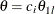andare displayed, where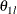andare the lower and upper alternative references, respectively.

For a two-sided design, the power is the probability of correctly rejecting the null hypothesis for the correct alternative. Thus, under the null hypothesis, the displayed power corresponds to a one-sided Type I error probability level—that is, the lowerlevel or the upperlevel.

The expected sample size as a percentage of the corresponding fixed-sample design is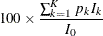where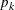is the stopping probability at stage k,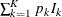is the expected information level, and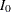is the information level for the fixed-sample design.

Sample Size Summary

When you use the SAMPLESIZE statement with the SEQDESIGN procedure, the Sample Size Summary table displays parameters for the sample size computation. It also displays the expected sample sizes or numbers of events for the model under both the null and alternative hypotheses.

The expected sample size is the average sample size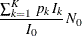whereis the stopping probability at stage k,is the expected information level,is the information level for the fixed-sample design, and
is the sample size for the fixed-sample design.

The expected number of events is the average number of events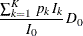where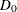is the fixed-sample number of events for the model.

Sample Size Information

The Sample Sizes (N) table displays the required sample sizes and information levels at each stage, in both fractional and integer numbers. The derived fractional sample sizes are under the heading Fractional N. These sample sizes are rounded up to integers under the heading Ceiling N. The matched integer sample sizes are also displayed for two-sample tests.

The Required Number of Events (D) table displays the required number of events required and information level at each stage.

The Number of Events (D) and Sample Sizes (N) table displays the number of events and sample size required at each stage with the study time. The derived times under the heading Fractional Time are not integers. These times are rounded up to integers under the heading Ceiling Time.

Stopping Probabilities

The Expected Cumulative Stopping Probabilities table displays the following information under each of the specified hypothetical references, whereare values specified in the CREF= option, andis the alternative reference:

• coefficientfor the hypothetical references. The valuecorresponds to the null hypothesis, andcorresponds to the alternative hypothesis

• expected stopping stage

• source of the stopping probability: reject(with STOP=REJECT or STOP=BOTH), accept(with STOP=ACCEPT or STOP=BOTH), or either reject or accept(with STOP=BOTH)

• expected cumulative stopping probabilities at each stage

For a one-sided design, the expected cumulative stopping probabilities under the hypothetical referencesare displayed.

For a two-sided design, the expected cumulative stopping probabilities under each of the hypothetical referencesandare displayed, whereandare the lower and upper alternative references, respectively.

Note that for a symmetric two-sided design, only the expected cumulative stopping probabilities under the hypothetical referencesare derived.

The expected stopping stage is given by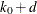, where the integer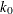and the fraction d (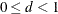) are derived from the expected information level equation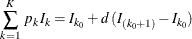whereis the stopping probability at stage k.

For equally spaced information levels, the expected stopping stage is reduced to the weighted average